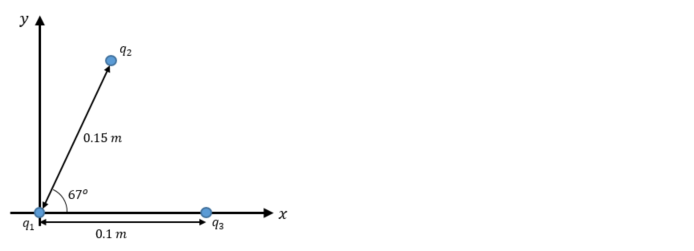# Problem: Find the magnitude and direction of the net electrostatic force on q 1 due to q 2 and q3 as shown in the figure. The charges are q 1 = +4.0 μC, q2 = -6.0 μC, q3 = -5.0 μC.

🤓 Based on our data, we think this question is relevant for Professor Stolbov's class at UCF.

###### Problem Details

Find the magnitude and direction of the net electrostatic force on q 1 due to q 2 and q3 as shown in the figure. The charges are q 1 = +4.0 μC, q2 = -6.0 μC, q3 = -5.0 μC.What scientific concept do you need to know in order to solve this problem?

Our tutors have indicated that to solve this problem you will need to apply the Coulomb's Law (Electric Force) concept. You can view video lessons to learn Coulomb's Law (Electric Force). Or if you need more Coulomb's Law (Electric Force) practice, you can also practice Coulomb's Law (Electric Force) practice problems.

What is the difficulty of this problem?

Our tutors rated the difficulty ofFind the magnitude and direction of the net electrostatic fo...as medium difficulty.

How long does this problem take to solve?

Our expert Physics tutor, Douglas took 6 minutes and 41 seconds to solve this problem. You can follow their steps in the video explanation above.

What professor is this problem relevant for?

Based on our data, we think this problem is relevant for Professor Stolbov's class at UCF.